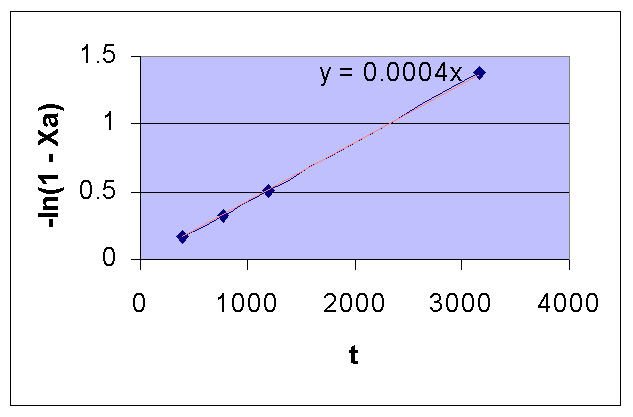### Test for First Order Reaction

The gas phase decomposition of A takes place according to the irreversible reaction, A → 3P. The kinetics of the reaction was studied by measuring the increase in pressure in a constant volume reaction vessel. At 504oC and an initial pressure of 312 mm Hg, the following data were obtained:

```Time (Sec)		390	777	1195	3155	∞
Total pressure (mm Hg) 	408	488	562	779	931
```
1. Test for a first order reaction.
2. Calculate the value of the specific reaction rate at 504oC

Calculations:

Total pressure of the system (π) and partial pressure of A (pA) are related as:

pA = pAo - (a/Δn)(π - πo)

where, πo is the initial pressure of the system;

pAo is the initial pressure of A;

a is the stoichiometric coefficient of A;

and Δn = stoichiometric coefficient of products minus reactants.

For the given problem, πo = pAo = 312 mm Hg (Assuming feed is pure A)

a = 1

Δn = 3-1 = 2

pA = 312 - (1/2)(π - 312)

i.e.,

pA = 312 - 0.5 (p - 312)

pA for various times are calculated as follows:

```Time (Sec) 	 	 	390	777	1195	3155	∞
Total pressure (mm Hg) 		408	488	562	779	931
pA (mm Hg) 			264	224	187	78.5	2.5
```

Fractional conversion of A is given by:

XA = (pAo - pA) / pAo

For various time values, XA and -ln(1- XA) is calculated and tabulated as follows:

```Time (Sec)			390 	 777	1195	3155	∞
pA (mm Hg) 			264 	 224 	187	78.5	2.5
XA				0.154	0.282	0.401	0.748	0.992
-ln(1 - XA)			0.167	0.331	0.512	1.378	4.828
```

For a first order reaction, a plot of t Vs. -ln(1 - XA) in a linear XY graph is a straight line with a slope of k.

From the following plot, it is seen that the given data is for a first order reaction.